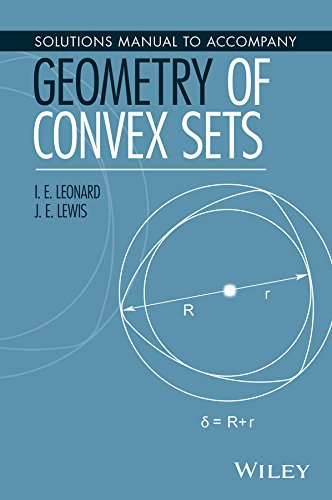HomeAbout us
AutoBabyBluetooth SpeakersClothingCombo KitsHomeLaser PrintersTextbooksToolsToysRunning ShoesSportsVideo ProjectorsWatches
Find it Cheaper# Solutions Manual to Accompany Geometry of Convex Sets - I. E. Leonard, Teacher's Edition, Paperback

John Wiley & Sons Inc
• Release Date   4/25/2016
• ISBN-13   9781119184188 | 978-1-119-18418-8
• ISBN   1119184185 | 1-119-18418-5
• Format   Paperback
• Author(s)   I. E. Leonard
• A Solutions Manual to accompany Geometry of Convex Sets Geometry of Convex Sets begins with basic definitions of the concepts of vector addition and scalar multiplication and then defines the notion of convexity for subsets of n-dimensional space. Many properties of convex sets can be discovered using just the linear structure. However, for more interesting results, it is necessary to introduce the notion of distance in order to discuss open sets, closed sets, bounded sets, and compact sets. The book illustrates the interplay between these linear and topological concepts, which makes the notion of convexity so interesting.Thoroughly class-tested, the book discusses topology and convexity in the context of normed linear spaces, specifically with a norm topology on an n-dimensional space.Geometry of Convex Sets also features:  An introduction to n-dimensional geometry including points. lines. vectors. distance. norms. inner products. orthogonality. convexity. hyperplanes. and linear functionals     Coverage of n-dimensional norm topology including interior points and open sets. accumulation points and closed sets. boundary points and closed sets. compact subsets of n-dimensional space. completeness of n-dimensional space. sequences. equivalent norms. distance between sets. and support hyperplanes · Basic properties of convex sets. convex hulls. interior and closure of convex sets. closed convex hulls. accessibility lemma. regularity of convex sets. affine hulls. flats or affine subspaces. affine basis theorem. separation theorems. extreme points of convex sets. supporting hyperplanes and extreme points. existence of extreme points. Krein–Milman theorem. polyhedral sets and polytopes. and Birkhoff’s theorem on doubly stochastic matrices Discussions of Helly’s theorem. the Art Gallery theorem. Vincensini’s problem. Hadwiger’s theorems. theorems of Radon and Caratheodory. Kirchberger’s theorem. Helly-type theorems for circles. covering problems. piercing problems. sets of constant width. Reuleaux triangles. Barbier’s theorem. and Borsuk’s problem Geometry of Convex Sets is a useful textbook for upper-undergraduate level courses in geometry of convex sets and is essential for graduate-level courses in convex analysis. An excellent reference for academics and readers interested in learning the various applications of convex geometry, the book is also appropriate for teachers who would like to convey a better understanding and appreciation of the field to students.I. E. Leonard, PhD, was a contract lecturer in the Department of Mathematical and Statistical Sciences at the University of Alberta. The author of over 15 peer-reviewed journal articles, he is a technical editor for the Canadian Applied Mathematical Quarterly journal.J. E. Lewis, PhD, is Professor Emeritus in the Department of Mathematical Sciences at the University of Alberta. He was the recipient of the Faculty of Science Award for Excellence in Teaching in 2004 as well as the PIMS Education Prize in 2002.Got an opinion on this product? Review it!